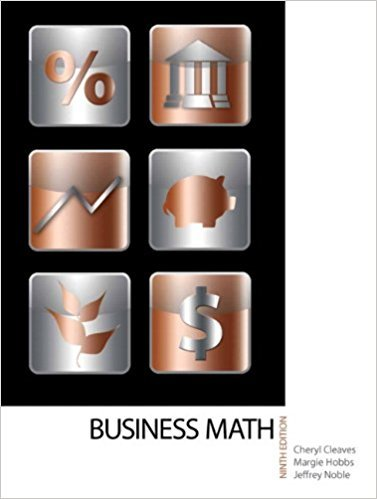×
×

# Solutions for Chapter 8-3: CASH DISCOUNTS AND SALES TERMS## Full solutions for Business Math, | 9th Edition

ISBN: 9780135108178Solutions for Chapter 8-3: CASH DISCOUNTS AND SALES TERMS

Solutions for Chapter 8-3
4 5 0 402 Reviews
12
3
##### ISBN: 9780135108178

Since 26 problems in chapter 8-3: CASH DISCOUNTS AND SALES TERMS have been answered, more than 17822 students have viewed full step-by-step solutions from this chapter. This textbook survival guide was created for the textbook: Business Math, , edition: 9. Business Math, was written by and is associated to the ISBN: 9780135108178. Chapter 8-3: CASH DISCOUNTS AND SALES TERMS includes 26 full step-by-step solutions. This expansive textbook survival guide covers the following chapters and their solutions.

Key Math Terms and definitions covered in this textbook
• Affine transformation

Tv = Av + Vo = linear transformation plus shift.

• Column space C (A) =

space of all combinations of the columns of A.

• Commuting matrices AB = BA.

If diagonalizable, they share n eigenvectors.

• Complete solution x = x p + Xn to Ax = b.

(Particular x p) + (x n in nullspace).

• Diagonalization

A = S-1 AS. A = eigenvalue matrix and S = eigenvector matrix of A. A must have n independent eigenvectors to make S invertible. All Ak = SA k S-I.

• Graph G.

Set of n nodes connected pairwise by m edges. A complete graph has all n(n - 1)/2 edges between nodes. A tree has only n - 1 edges and no closed loops.

• Hilbert matrix hilb(n).

Entries HU = 1/(i + j -1) = Jd X i- 1 xj-1dx. Positive definite but extremely small Amin and large condition number: H is ill-conditioned.

• Least squares solution X.

The vector x that minimizes the error lie 112 solves AT Ax = ATb. Then e = b - Ax is orthogonal to all columns of A.

• Left inverse A+.

If A has full column rank n, then A+ = (AT A)-I AT has A+ A = In.

• Left nullspace N (AT).

Nullspace of AT = "left nullspace" of A because y T A = OT.

• Length II x II.

Square root of x T x (Pythagoras in n dimensions).

• Markov matrix M.

All mij > 0 and each column sum is 1. Largest eigenvalue A = 1. If mij > 0, the columns of Mk approach the steady state eigenvector M s = s > O.

• Multiplication Ax

= Xl (column 1) + ... + xn(column n) = combination of columns.

• Nilpotent matrix N.

Some power of N is the zero matrix, N k = o. The only eigenvalue is A = 0 (repeated n times). Examples: triangular matrices with zero diagonal.

• Norm

IIA II. The ".e 2 norm" of A is the maximum ratio II Ax II/l1x II = O"max· Then II Ax II < IIAllllxll and IIABII < IIAIIIIBII and IIA + BII < IIAII + IIBII. Frobenius norm IIAII} = L La~. The.e 1 and.e oo norms are largest column and row sums of laij I.

• Normal matrix.

If N NT = NT N, then N has orthonormal (complex) eigenvectors.

• Polar decomposition A = Q H.

Orthogonal Q times positive (semi)definite H.

• Row picture of Ax = b.

Each equation gives a plane in Rn; the planes intersect at x.

• Spectral Theorem A = QAQT.

Real symmetric A has real A'S and orthonormal q's.

• Volume of box.

The rows (or the columns) of A generate a box with volume I det(A) I.

×